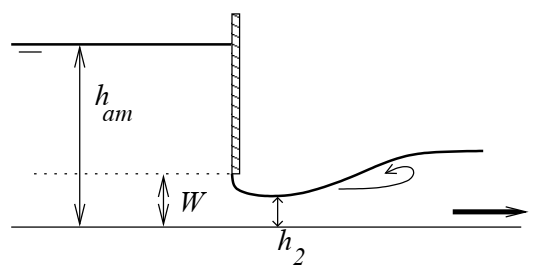# Free flow sluice gateExcerpt from Baume, J.-P., Belaud, G., Vion, P.-Y., 2013. Hydraulique pour le génie rural, Formations de Master, Mastère Spécialisé, Ingénieur agronome. UMR G-EAU, Irstea, SupAgro Montpellier.

$$W$$ is the gate opening, $$h_{am}$$ the upstream water level and $$L$$ the date width. The stage-discharge equation of the free flow sluice gate is derived from Bernoulli's load conservation relationship between the upstream side of the valve and the contracted section.

The height $$h_2$$ corresponds to the contracted section and is equal to $$C_c W$$ where $$C_c$$ is the contraction coefficient. The stage-discharge equation is often expressed as a function of a flow coefficient $$C_{d}$$ in the form of:

$$Q = C_d L W \sqrt{2g} \sqrt{h_{am}}$$

So we have the relationship between $$C_d$$ and $$C_c$$ :

$$C_d = \frac{C_c}{\sqrt{1 + C_c W / h_{am}}}$$

Numerous experiments were conducted to evaluate $$C_d$$, which varies little around 0.6. As a first approximation, for a low $$W / h_{am}$$ (undershot gate, most frequent case), $$C_d$$ is close to $$C_c$$ and can be chosen equal to 0.6.

Discharge coefficients $$C_d$$ are given by abacuses, which can be found in specialized books if necessary. They range from 0.5 to 0.6 for a vertical sluice gate, from 0.6 to 0.7 for a radial gate, up to 0.8 for an inclined sluice gate.